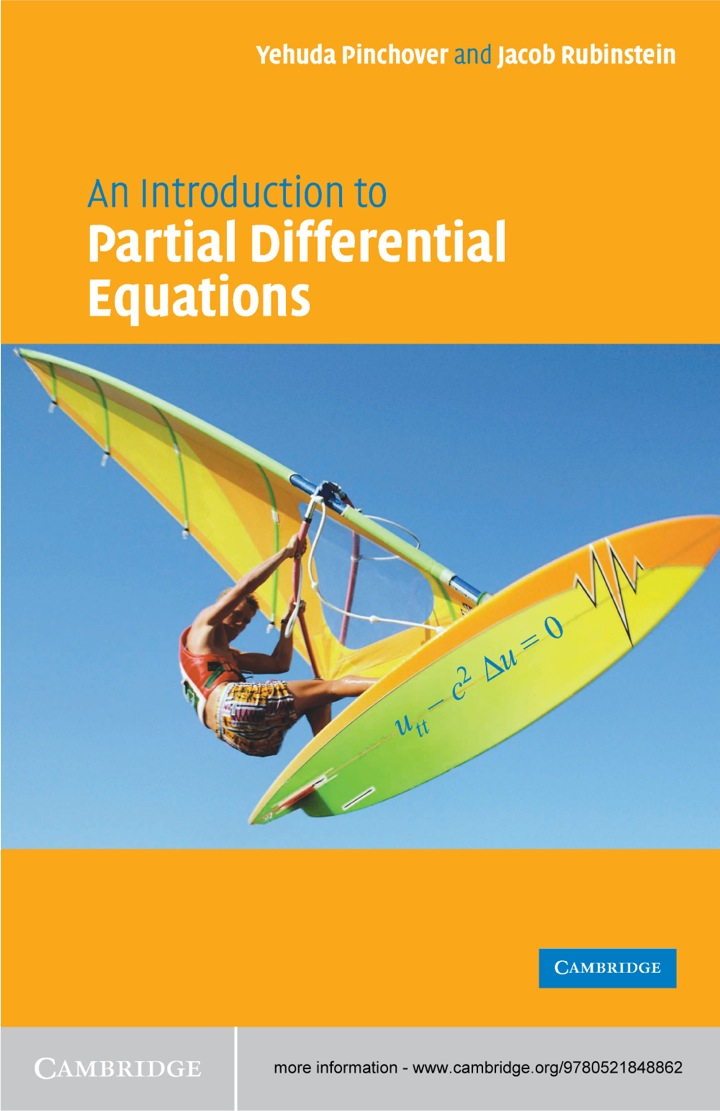# An Introduction to Partial Differential Equations

## An Introduction to Partial Differential Equations

By Yehuda Pinchover; Jacob Rubinstein

Expires on May 15th, 2022
\$78

Publisher List Price: \$0.00

A complete introduction to partial differential equations, this textbook provides a rigorous yet accessible guide to students in mathematics, physics and engineering. The presentation is lively and up to date, paying particular emphasis to developing an appreciation of underlying mathematical theory. Beginning with basic definitions, properties and derivations of some basic equations of mathematical physics from basic principles, the book studies first order equations, classification of second order equations, and the one-dimensional wave equation. Two chapters are devoted to the separation of variables, whilst others concentrate on a wide range of topics including elliptic theory, Green's functions, variational and numerical methods. A rich collection of worked examples and exercises accompany the text, along with a large number of illustrations and graphs to provide insight into the numerical examples. Solutions to selected exercises are included for students whilst extended solution sets are available to lecturers from solutions@cambridge.org.

Subject: Mathematics & Statistics -> Mathematics -> Mathematics General1st edition
Publisher: Cambridge University Press 5/12/05
Imprint: Cambridge University Press
Language: English

ISBN 10: 1139637258
ISBN 13: 9781139637251
Print ISBN: 9780521613231

Live Chats